# Pre-Algebra : Negative Numbers

## Example Questions

1 2 3 4 5 6 7 8 10 Next →

### Example Question #81 : The Number System

Solve: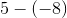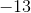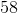Explanation:

When subtracting with a negative sign, it turns into addition. Now we have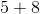. The answer is### Example Question #91 : The Number System

Solve: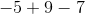Explanation:

Let's work from left to right since it's only subtraction and addition. Sinceis greater thanand it's positive our answer is positive, but we treat this like a subtraction problem because theis negative.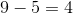.  Now we have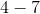is greater thanand it's negative so our answer is negative and we treat this like a subtraction problem.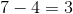, but since our answer should be negative our answer is### Example Question #92 : Negative Numbers

Solve: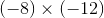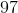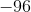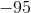Explanation:

When multiplying two negative numbers, the negative signs cancel each other out, making for a positive solution. If only one number is negative, the solution will be negative.

Therefore we can rewriteas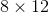, which is.

### Example Question #91 : Negative Numbers

Solve: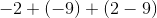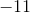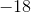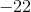Explanation:

Solve by first combining signs and eliminate the parentheses.

A positive multiplied with a negative sign will give a negative sign.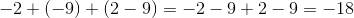### Example Question #93 : Negative Numbers

Solve: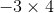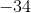Explanation:

First, since we are multiplying a negative value with a positive value, we need to realize the product is going to be negative. Next, we can multiply the values.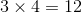Since the answer is negative, the answer is.

### Example Question #91 : Negative Numbers

Solve: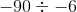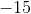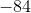Explanation:

When dividing with two negative values, the answer becomes positive. Then we just divideby, which equals. The answer is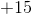or### Example Question #10 : Divide Integers: Ccss.Math.Content.7.Ns.A.2b

Solve: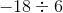Explanation:

When dividing with negative numbers, we count the number of negative numbers. We have one so that means the answer is negative. So the quotient is### Example Question #1 : Multiply And Divide Rational Numbers Using Properties Of Operations: Ccss.Math.Content.7.Ns.A.2c

Solve: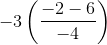Explanation:

When solving this problem remember our orders of operation, or PEMDAS.

PEMDAS stands for parentheses, exponents, multiplication/division, and addition/subtraction. When you have a problem with several different operations, you need to solve the problem in this order and you work from left to right for multiplication/division and addition/subtraction.

Simplify the quantities inside the parentheses.  Then multiply and distribute the coefficient in front.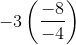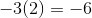### Example Question #1 : Multiply And Divide Rational Numbers Using Properties Of Operations: Ccss.Math.Content.7.Ns.A.2c

Solve: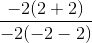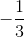Explanation:

When solving this problem remember our orders of operation, or PEMDAS.

PEMDAS stands for parentheses, exponents, multiplication/division, and addition/subtraction. When you have a problem with several different operations, you need to solve the problem in this order and you work from left to right for multiplication/division and addition/subtraction.

Evaluate the quantities inside the parentheses first.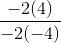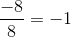1 2 3 4 5 6 7 8 10 Next →

### All Pre-Algebra Resources